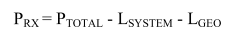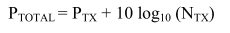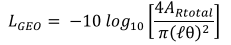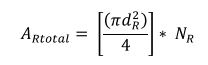Hello everyone,Lsystem (system loss) is set to 8dB. PTotal can be calculated as:where Ntx (number of receiver) = 1 and PTx (transmitted power) =7.78 dBm. LGeo can be calculated as:where d2R (receiver diameter) = 0.07, l=1, θ (divergence angle) =0.05 and Nr (number of receiver) =1.

The problem is, I got received power=10.72 dBm which is illogical value. As I know, the received power must be lower than transmitted power.
I hope that anyone may help me to understand this situation.
For your information, I refer this paper for the calculation: https://ieeexplore.ieee.org/document/6015903
Thank you.

#### Attachments

trurle
You have above the θl<d2R, which is outside of the formula applicability range.
Your beam is not illuminating the entire receiver area, therefore the error.

•tech99
You have above the θl<d2R, which is outside of the formula applicability range.
Your beam is not illuminating the entire receiver area, therefore the error.
What is the applicable range?

trurle
What is the applicable range?
Range (all values) of input variables producing a valid output.

•